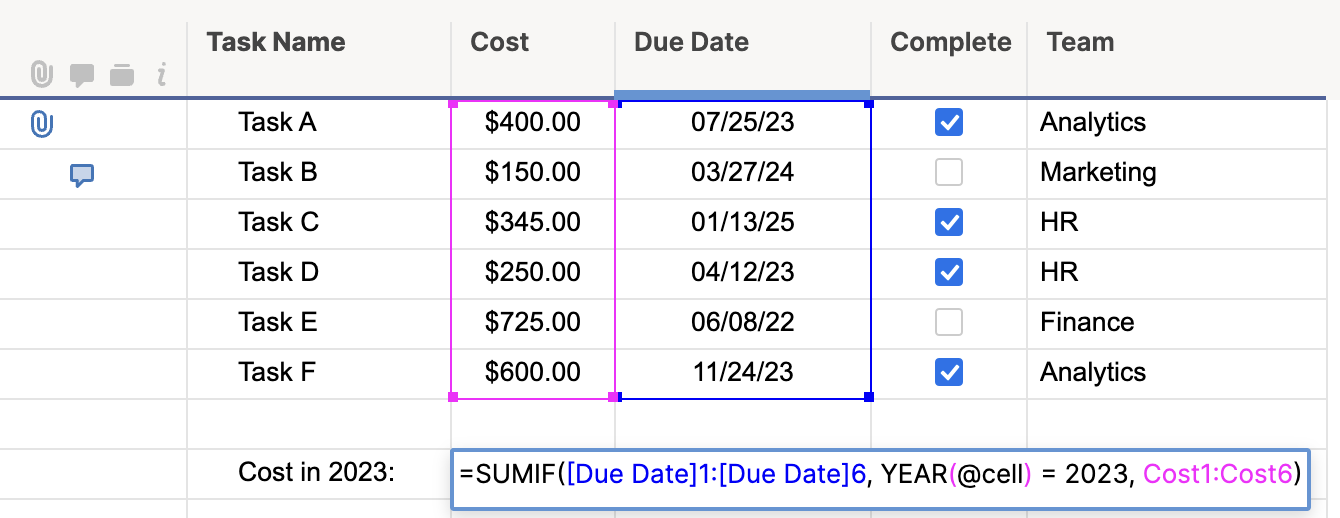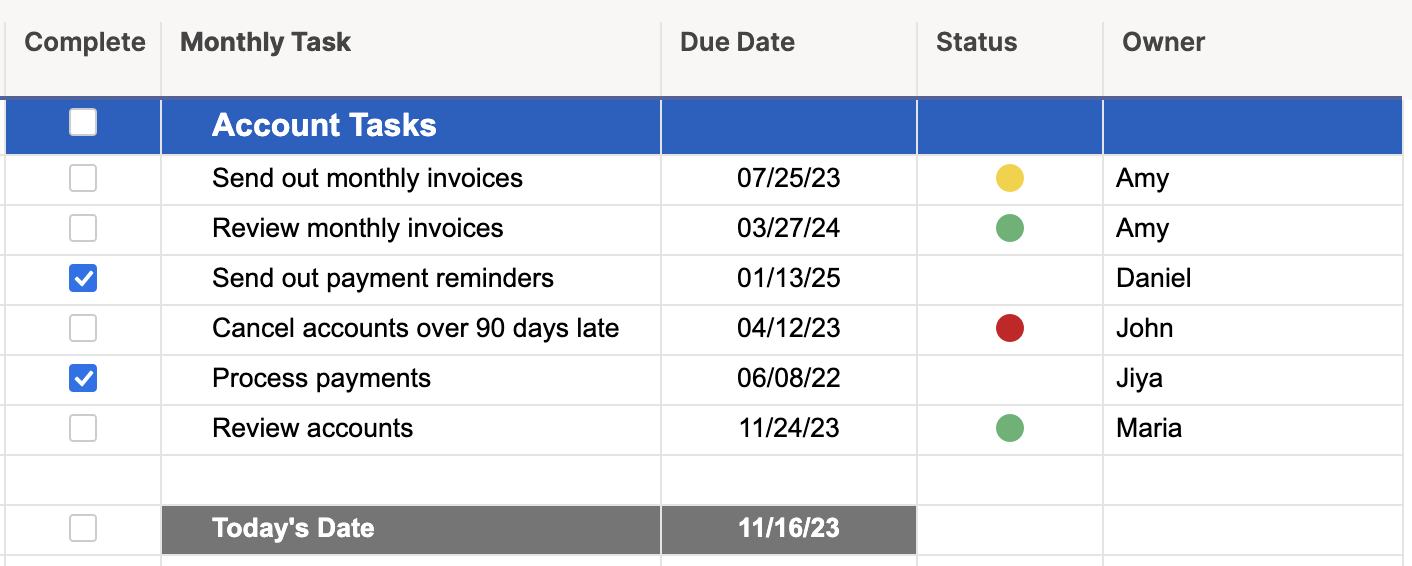Smartsheet
• Pro
• Enterprise

## Capabilities

### Who can use this capability

Users that are the sheet Owner or have Admin sharing permissions can create and edit formulas on a sheet. Users with Editor sharing permissions can also create and edit formulas in unlocked cells on a sheet.

# Create efficient formulas with @cell and @row

Optimize your formulas to improve the overall performance of your sheet and prevent having to manually reference cells in formulas.

• Smartsheet
• Pro
• Enterprise

## Permissions

Users that are the sheet Owner or have Admin sharing permissions can create and edit formulas on a sheet. Users with Editor sharing permissions can also create and edit formulas in unlocked cells on a sheet.

As your sheets get larger, having efficiently created formulas becomes more helpful to their performance. Here’s a look at two functions that will help make your formulas more efficient, @cell and @row.

### Use @cell to perform smarter cell calculations

When you want to perform calculations in formulas that look at ranges of cells, for example: SUMIF, SUMIFS, COUNTIF, and COUNTIFS, you can use the @cell argument in the criteria of the function. The @cell argument performs a calculation on each row at the same time that the primary function (SUMIF for example) is evaluating the criteria in the range, making your formula more efficient.

For example, using the sample data in the image below, if you wanted to sum the cost of all tasks whose due date occurred in 2023, you would create the following formula:

=SUMIF([Due Date]1:[Due Date]5, YEAR(@cell) = 2023, Cost1:Cost5)Add the IFERROR function to your formula to ensure that you don't get an error if @cell encounters a blank cell in the formula. For example: =COUNTIF(Date:Date, IFERROR(YEAR(@cell), 0) = 2016)

### Use @row to improve formula performance

With @row, you can improve performance with the automatic changing of cell references in a column (for example, the status of your tasks) for a large number of rows.
Let’s say we want to use a formula to change symbols in the status column of this grid automatically:Here’s an example of a formula you might build to automate the RYG ball color change in your Status column:

=IF(Complete3 <> 1, IF(TODAY() - [Due Date]3 > 0, "Red", IF(TODAY() - [Due Date]3 > -3, "Yellow", "Green")))

The above formula contains multiple references to cells on row 3. Adding a row above row 3, results in Smartsheet automatically updating all of the cell references in the formula to row 4. Smartsheet would also modify formulas in every row below in the same fashion. The more formulas that need to be modified, the more your performance in Smartsheet becomes impacted.

To possibly improve performance in your sheets, substitute the row numbers with @row in your cell references. This is how the formula would look using @row:

=IF(Complete@row <> 1, IF(TODAY() - [Due Date]@row > 0, "Red", IF(TODAY() - [Due Date]@row > -3, "Yellow", "Green")))

Smartsheet won’t need to modify the cell references if the row is moved—resulting in quicker sheet load and save times. You can also copy and paste this formula without having to manually change the row numbers on cell references, saving you time when you need to copy your formulas.

#### Still need help?

Use the Formula Handbook template to find more support resources, and view 100+ formulas, including a glossary of every function that you can practice working with in real time, and examples of commonly used and advanced formulas.

Find examples of how other Smartsheet customers use this function or ask about your specific use case in the Smartsheet online Community.

YesNo